International Journal of Geomagnetism and Aeronomy
Vol 2, No. 2, August 2000

# A hodograph method of analysis for geomagnetic pulsation observations

V. A. Pilipenko and Yu. P. Kurchashov

Institute of Physics of the Earth, Moscow, Russia

### Abstract

A new method for the determination of the frequency of magnetospheric Alfvén resonances from ground-based geomagnetic pulsation measurement data is proposed. Unlike the standard gradient method, the proposed approach is based on calculations of a hodograph of the complex signal spectra ratio at two stations. Hodograph data analysis makes it possible to obtain more information on parameters of the magnetospheric resonator with simultaneous monitoring of stability of the estimates obtained. The hodograph method is experimentally evaluated using the Pc4 pulsation data recorded at a meridional network of midlatitude stations.

### Introduction

Hydromagnetic diagnostics of the magnetospheric plasma density from ground-based data is based on signatures of the resonance effects in the spatial-spectral structure of the geomagnetic pulsation field. The gradient method [Baranskiy et al., 1985; Best et al., 1986] seems to be the most effective method for isolation of resonance frequencies in the spectrum of geomagnetic pulsations. This method is based on precision measurements of the H components of the pulsation field at two stations situated at a small spacing along the geomagnetic meridian. The physical basis of the gradient method is the characteristic feature of the spatial structure of the pulsation field in the resonance region, notably the superposition of the large-scale magneto-acoustic wave from the source and the local resonance response of the magnetospheric Alfvén resonator. It is the difference in spatial scales of these wave processes that provides the basic possibility of isolation of even feeble resonance effects. The gradient method has successfully been used for the determination of parameters of the magnetospheric resonator at middle and low latitudes from Pc3-4 pulsation data [Baranskiy et al., 1988; Fedorov et al., 1989; Pilipenko et al., 1988]. A modified gradient method ensures the determination of resonance magnetospheric frequencies even in the presence of lateral geoelectric inhomogeneity of the crust [Pilipenko and Fedorov, 1993]. To determine the resonance frequency of the field line running between the stations, the standard method uses either the ratio of the signal amplitude spectra [Baranskiy et al., 1985] or the difference of the phase spectra [Best et al., 1986; Waters et al., 1991].

In the present paper, we propose a geometric method for analyzing gradient measurement data, which simultaneously uses amplitude and phase information. To evaluate the new method, we used Pc4 pulsation data from a meridional network of magnetic stations in the L range from 4.7 to 3.0 [Green, 1978]. This event can be considered a reference event since it had received thorough study [Baranskiy et al., 1988; Fedorov et al., 1989] to demonstrate the resonance magnetospheric effects.

According to resonance theory (see the review by Pilipenko and Fedorov  and references therein), the spectrum of the resonant component of a signal can be presented in the form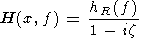(1)

where z = (x-xR(f))/e is the normalized distance from the resonance point xR(f) ; hR(f) is the amplitude of pulsations at the resonance point, x is the field line coordinate measured along the geomagnetic meridian on the ground surface, and e is the half width of the resonance region. The x axis is directed from the source of oscillations. (It is assumed that the resonance frequency fR(x) increases with x and e >0 ). By measuring signal's resonant component at two stations of a meridional profile with coordinates x1 and x2 one can find the ratio of their complex spectra: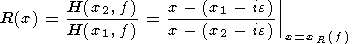(2)

Upon a change in frequency f, the image R{x(f)} will cover on the complex plane a certain curve which is a hodograph of the ratio of the spectra. This hodograph has a number of remarkable properties that make it a quite suitable and informative tool for data representation. For the time being, we will not be interested in specific behavior and range of values of xR(f) we will just assume that x=xR covers the entire real axis (Figure 1a). The right-hand part of formula (2) essentially prescribes a fractional linear transformation of the complex plane  x. Such a transformation translates a set of straight lines and circle into the very same set. The pole (x2 - i e, e >0) lies below the real axis;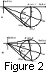therefore it is transformed into a circle (Figure 2a), and in this context, with increasing x, its image circles this circle counterclockwise. Note that the circle does not contain an origin point 0, since zero and pole of (2) the mapping lie on one side of the real axis. As is seen from Figure 1a, the spectrum ratio argument arg  R equal to the angle of rotation from the vector representing the denominator to the numerator vector does not reverse its sign upon a change in x. From this and from the fact that R)=1, it follows that the hodograph is tangent to the real axis at point R=1. The half plane, wherein lies the hodograph, is determined by the signal phase difference between the two stations and by the choice of their ordering in calculating the cross spectra. If the signal at a station whose spectrum is in the numerator of (2) is lagging in phase behind the other station, then the hodograph is below the real axis.

Let us place on the hodograph a coordinate scale containing certain characteristic values of x. Let x0 be the coordinate of the point that lies exactly in the middle between the stations (x0 =(x1 + x2)/2 ). As is seen from Figure 1a, for a random pair of points x and x' symmetric in relation to x0 , the arguments of corresponding values of R and R' are the same, and the moduli are mutually inverse. This means that the images of these points lie on one secant drawn from the origin 0 (Figure 2a). In particular, this goes for the coordinates of both observatories, x1 and x2. The minimum and maximum values of R are also seen to be symmetric relative to x0. Symmetric points are confluent when x=x'=x0 whereas their images merge when the secant changes into a tangent. Therefore the image of x0 lies on the tangent to the hodograph, drawn from origin 0.

To completely prescribe the circle, its radius r remains to be determined. Let 2 d denote the distance x2-x1 between the stations, and comparing Figures 1b and 2a, we find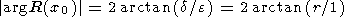(3)

In other words, the angle arg R(x0), at which the hodograph is seen from the origin of coordinates, is equal to the angle at which the interval between the stations is seen from the distance equal to a half width of the resonance. From (3), we find the hodograph radius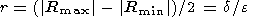(4)

where Rmin and Rmax are the minimum and maximum spectrum ratio values that are attained at points xmin and xmax. Thus the hodograph radius is equal to the distance between the stations expressed in units of the resonance width. This is a clearly visual criterion for determining a situation: large hodographs correspond to narrow resonances or distant stations.

It is seen from Figure 1a that the triangle formed by the numerator and denominator of R at x=x2 differs from half of a similar triangle at x=x0 by 2 times increased cathetus d This fact represented in Figure 2a by a doubled cathetus r in the triangle with vertices 0 and 1. This means that the image of the coordinate x2 is diametrically opposite to the image of an infinite point(R=1) and that at x=x2 there is an extremum of the imaginary part of the spectrum ratio Im  R.

Let us note that the secant that corresponds to the minimum and maximum values of R(f) passes through the hodograph center, whereas the secant corresponding to the positions of the stations passes through the end of diameter connecting R(x2) and 1 (Figure 2a). This means that the interval between the points xmin and xmax contains within itself the interval between the stations. With a monotonic dependence xR(f), from this it follows that the resonance frequencies of the stations always lie between the frequencies corresponding to Rmin and Rmax.

Heretofore, we discussed the hodograph orientation with increasing  x. This orientation coincides with the orientation with increasing f if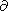xR /f >0 and is opposite otherwise. Upon a reversal of sign of the resonance frequency gradient, the sign of e also reverses, which in turn results in a reversal of the sense of covering the hodograph. Thus the hodograph frequency scale is always counterclockwise.

As was noted by Gul'el'mi , having simultaneously available frequency dependencies of the amplitude ratio and phase differences at two stations, one can find a continuous dependence of the resonance frequency on the coordinate fR(x). This dependence can also be reconstructed in our approach. To this end, we will use the circle that on the one hand, the spectrum ratio R(x) is determined by formula (2) as a function of coordinate x, and on the other hand, this ratio is known from experiment as a tabulated frequency function. In fact, R(x) is a reversible function of the normalized coordinate X= (x1 -x2)/e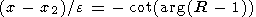(5)

Using relationship (5), one can reconstruct the dependence of resonance frequency on coordinate.

In applications of the gradient method to actual data, there were situations when the relationship Rmin Rmax =1 was not met. A possible cause was assumed to be the effect of local geology displaying itself in the occurrence of amplitude and phase distortions specific to each station. To exclude these distortions, Green et al.  proposed a modified gradient method based on the assumption of independence of these distortions of frequency. In our approach, within the assumptions of the modified gradient method, the complex spectrum ratio acquires an additional constant complex factor  M . This results in expansion (contraction) of the hodograph at a coefficient M and its rotation at angle arg M relative to the origin of coordinates (Figure 2b). Neither the linear element ratio nor the angular lengths of arcs between the characteristic points of the hodograph are affected by this transformation; therefore the majority of the aforementioned hodograph properties still hold true. The basic difference is that the hodograph is no longer tangent to the real axis at R=1. Instead, R=M will be the image of x=. The sign of phase lagging between the stations is no longer determined unambiguously by the half plane wherein the hodograph is found. However, the phase difference sign can be determined by the behavior of arg R(x). For example, if rotation from R) to R(x0) proceeds clockwise, then the phase difference, after geology correction, is negative. Also lost is the extremal property of Im  R for the point representing one of the stations. In order to determine the value of (  d / e ) from (4), one now must use, instead of the hodograph radius r, its "modified" value (r/ M ). In addition, the term ( R-M ) will replace ( R-1 ) in (5). Since in approaching R(), the conditions of applicability of initial assumptions are violated and experimental points drift from the circle, point R=M may not lie on the real hodograph. However, to determine M, one can use the evident relationships (Figure 2b)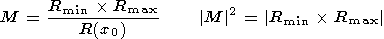(6)

As experience has shown, even an intuitive visual extrapolation of that part of the hodograph which approximates a circle quite well can give a reliable estimate of this coefficient.

The geometric method of analysis of gradient observations is advantageous in that it makes possible a preliminary best-fit approximation for a certain set of frequencies, after which, instead of individual values of R(f) subject to random deviations, one can take values closest thereto that belong to this idealized hodograph. Selection of the frequency range to be used for the construction of the best-fit approximation circle is governed by the following two circles: (1) frequencies far away from the resonance region clearly do not fit the idealized hodograph and are interferences; (2) isolation of a too narrow frequency range within the resonance region makes the hodograph parameters too sensitive to a particular selection of the initial and final frequencies. In practice, estimates of the parameters are stable enough when the selected frequency range turns out to be of the same order of magnitude as the difference of frequencies corresponding to Rmin and Rmax. If the distance between the stations is known, then one can, switching from normalized to conventional coordinates and using the ratio (  d / e ) defined from the radius of best-fit hodograph obtain the resonance width and the Q factor of the resonator.

### Experimental Evaluation

Oscillations in the Pc4 range were detected at five stations of a United Kingdom meridional network: (1) (  F =62.5o ), (2) (  F =60.0o ), (3) ( F =58.9o ), (4) ( F =57.1o ), and (5) ( F =54.6o ). The extreme northern and southern stations had a rather low signal; therefore, data from these stations should be treated with caution. Amplitude and phase spectra at each station were calculated using the Fourier transform and then smoothed with the aid of a five-point running average. The network of stations is described in detail by Green , and a general view of the spectra can be found in the papers by Baranskiy et al.  and Fedorov et al. .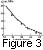The spectrum ratio hodographs were calculated using a specially developed program making it possible to interactively determine the values of resonance frequencies f1= fR(x1), f2= fR(x2), fA= fR(x0), and the Q factor. Stability of the results obtained was monitored by (1) varying the length of the spectral interval wherein appropriate points fit the circle well and (2) changing to the inverse ratio R-1 (f). Figure 3 shows the results of calculations of resonance frequencies fRF ) for each pair of stations. For comparison, Figure 3 shows by circles the values of resonance frequencies fAF) of the field lines running between the stations, determined by the standard gradient method [Baranskiy et al., 1988]. This method is seen to give somewhat underestimated (by 1 mHz on average) values of local resonance frequencies. This discrepancy is attributed to the fact that Baranskiy et al.  did not introduce in their calculations the amplitude and phase correction coefficients amounting for this profile to M =0.90.2 and arg M=105o.

It should be noted that the resonance frequencies of a particular station, calculated by the hodograph method in tandem with a northern or southern station, differed on average by 0.2-0.5 mHz. This difference (clearly seen in Figure 3 between greatly spaced stations) displays itself in small jumps in fRF ) at latitudes of the stations. This discrepancy seems to be associated with the occurrence of asymmetry of the resonance amplitude curve in receding from the resonance point: the northern slope becomes steeper than the southern one, which is ignored by the main leading (1) of the asymptotic expansion of the wave field in the resonance region [Fedorov et al., 1989].As was mentioned above, the described approach makes it possible not only to determine the discrete values of resonance frequencies at characteristic points, but also to reconstruct the continuous latitudinal distribution of fRF ). Figures 4a and 4b show examples of restoration of the resonant frequency dependence fR(X) on the normalized distance X=(x-x2)/e for station pairs 2/3 and 3/4. The station positions correspond to points X=0 and X=(x1-x2)e, the latter is being marked by vertical bar. Note that the hodograph method enables us to restore the distribution fR(X) not only between the stations, but even in a latitude range beyond this interval.

As was noted above, the hodograph method also makes it possible to determine the QA factor of the magnetospheric Alfvén resonator. It should be kept in mind that the Q factor of Alfvén oscillations observed on the ground comprises the contribution of the effects of phase mixing and spatial integration [Yumoto et al., 1995]. The magnetospheric resonator Q factor values QA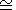63 calculated considering this circumstance correspond to the values of a model of the ionospheric dissipation of the fundamental mode of Alfvén field line oscillations [Yumoto et al., 1995].

### Conclusions

A visual geometric method of analysis of pulsation observations at a meridional profile of stations has a number of advantages compared with the standard gradient method. The new approach based on the construction of a hodograph of complex spectrum ratio allows a visual check of the degree of fit of experimental data to a theoretical model in the entire frequency range. Accordingly, calculations of parameters of the resonance structure are carried out by least-square approximation of a curve in a finite frequency range and not by one characteristic point of the spectrum, as in the gradient method. As a result, the results produced by the hodograph method turn out to be more stable. Besides, this method has additional potentialities compared with the standard gradient method. Measurements at two points can provide the following: (1) not only the resonance frequency of the median field line, but also local resonant frequencies of the observation points themselves; (2) the Q factor of the magnetospheric Alfvén resonator; and (3) continuous distribution of resonance frequencies in a finite latitude range beyond the latitudes of the observation points.

#### Acknowledgments

The authors thank L. N. Baranskiy and C. Green for making available experimental data. This work was supported by the Russian Foundation for Basic Research (grant N 95-05-17562).

### References

Baranskiy, L. N., Yu. E. Borovkov, M. B. Gokhberg, and S. M. Krylov, A gradient method of measurement of resonance frequencies of field lines, Izv. Akad. Nauk SSSR Fiz. Zemli (in Russian), 8, 74, 1985.

Baranskiy, L. N., S. P. Belokris, Yu. E. Borovkov, M. B. Gokhberg, E. N. Fedorov, and C. A. Green, A gradient method of reconstruction of the meridional structure of geomagnetic pulsation field, Dokl. Akad. Nauk SSSR (in Russian), 229, 1347, 1988.

Best, A., S. M. Krylov, Yu. P. Kurchashov, and V. A. Pilipenko, A gradient temporal analysis of the Pc3 pulsations, Geomagn. Aeron. (in Russian), 26 (6), 980, 1986.

Fedorov, E. N., et al., Diagnostics of the magnetospheric plasma density from gradient observations of geomagnetic pulsations, Izv. Akad. Nauk SSSR Fiz. Zemli (in Russian), 12, 63, 1989.

Green, A. W., E. W. Worthington, L. N. Baransky, E. N. Fedorov, N. A. Kurneva, V. A. Pilipenko, D. N. Schvetzov, A. A. Bektemirov, and G. V. Philipov, Alfvén field line resonances at low latitudes ( L=1.5 ), J. Geophys. Res., 98A (9), 15,693 1993

Green, C., Meridional characteristics of Pc4 micropulsations event in the plasmasphere, Planet. Space Sci., 39, 569, 1978.

Gul'el'mi, A. V., Hydromagnetic diagnostics and geoelectric prospecting, Usp. Fiz. Nauk (in Russian), 158, 605, 1989.

Pilipenko, V. A., and E. N. Fedorov, Magnetotelluric sounding of the crust and hydromagnetic monitoring of the magnetosphere with the use of ULF waves, Annali di Geofisica, 36 (5-6), 19, 1993.

Pilipenko, V. A., T. A. Povzner, I. V. Savin, and Ya. S. Nikomarov, Local spatial structure of the geomagnetic pulsation field at middle latitudes, Izv. Akad. Nauk SSSR Fiz. Zemli (in Russian), 10, 54, 1988.

Yumoto, K., V. Pilipenko, E. Fedorov, N. Kurneva, and K. Shiokawa, The mechanisms of damping of geomagnetic pulsations, J. Geomag. Geoelectr., 47 (2), 163, 1995.

Waters, C. L., F. W. Menk, and B. J. Fraser, Phase structure of low latitude Pc3-4 pulsations, Planet. Space Sci., 39, 569, 1991.

Load files for printing and local use. This document was generated by TeXWeb (Win32, v.2.0) on August 5, 2000.